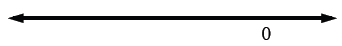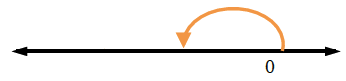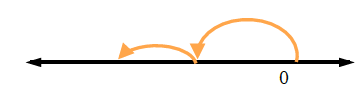### Home > MC1 > Chapter 2 > Lesson 2.4.1 > Problem2-132

2-132.

Simplify each expression below. For each expression, draw a picture or show how you know your answer makes sense.

1.  $5+(−4)+12$

$5+(−4)+12=13$
Remember to show how you know.

1.  $6(−2)+10$

$6(−2)+10=−2$
Remember to show how you know.

1.  $100(−5)$

$100(−5)=−500$
Remember to show how you know.

1.  $−212+(−102)$

$−212$

$+ \left(−102\right)$1.  $4+6(3)+2(5−1)$

Remember to use the order of operations.
Refer to the Math Notes box in Lesson 2.4.1 for a reminder.

1.  $5+3(5)−4(5)$

Simplify: $5+15−20=$ ?